You can Download Samacheer Kalvi 12th Maths Book Solutions Guide Pdf, Tamilnadu State Board help you to revise the complete Syllabus and score more marks in your examinations.

## Tamilnadu Samacheer Kalvi 12th Maths Solutions Chapter 10 Ordinary Differential Equations Ex 10.1

Question 1.
For each of the following differential equations, determine its order, degree (if exists)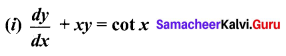Solution:
Order = 1,
Degree = 1

(ii)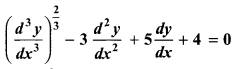Solution: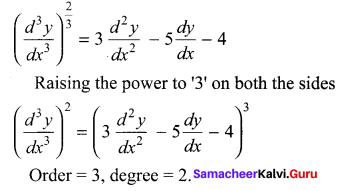(iii)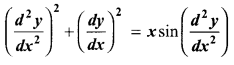Solution: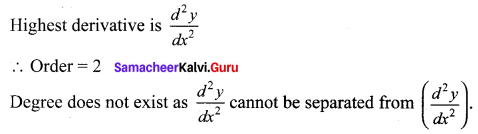(iv)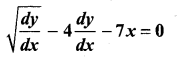Solution: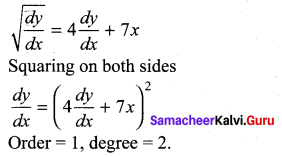(v)Solution: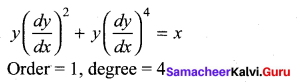(vi)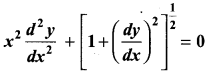Solution: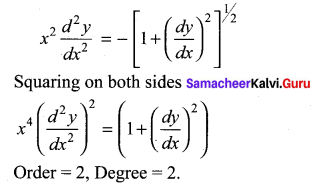(vii)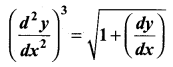Solution: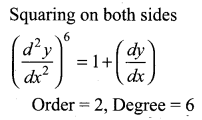(viii)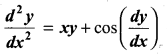Solution:
Order = 2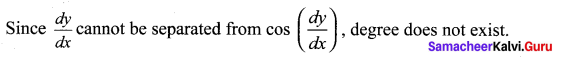(ix)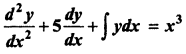Solution: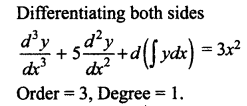(x)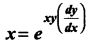Solution: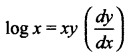Order = 1,
degree = Not exist### Samacheer Kalvi 12th Maths Solutions Chapter 10 Ordinary Differential Equations Ex 10.1 Additional Problems

Question 1.
Find the order and degree of the following differential equations: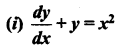Solution:
Order = 1,
Degree = 1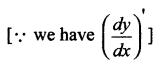(ii) y’ + y2 + y3 = 0
Solution:
Order = 1,
Degree = 1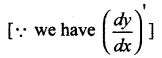(iii)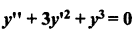Solution: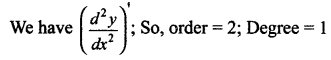(iv)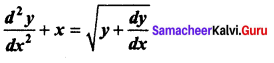Solution: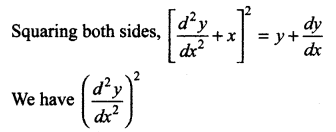∴ Order = 2
Degree = 2

(v)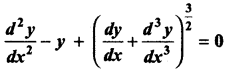Solution: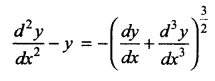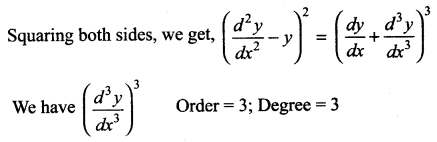(vi)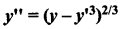Solution: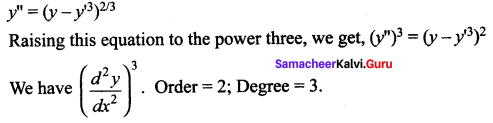(vii)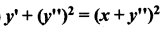Solution: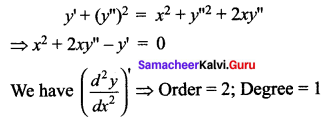(viii)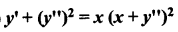Solution: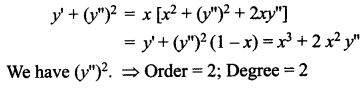(ix)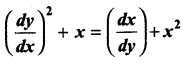Solution: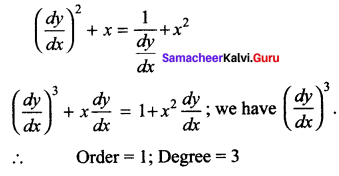(x) sin x(dx + dy) = cos x(dx – dy)
Solution:
Order = 1;
Degree = 1